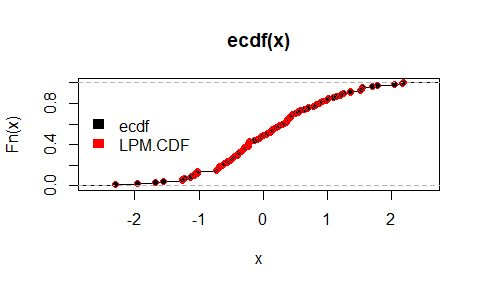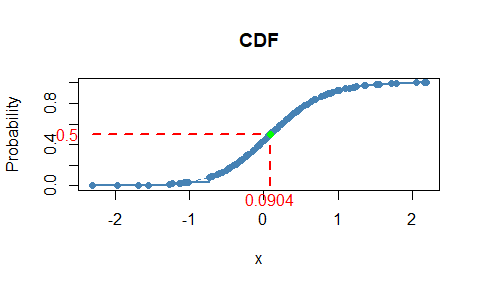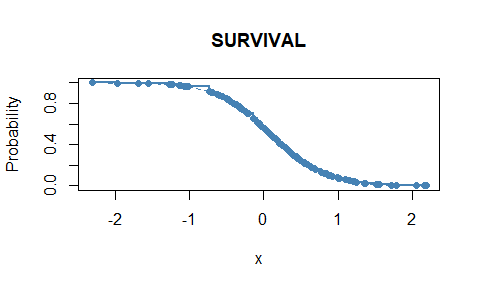# Partial Moments

Why is it necessary to parse the variance with partial moments? The additional information generated from partial moments permits a level of analysis simply not possible with traditional summary statistics.

Below are some basic equivalences demonstrating partial moments role as the elements of variance.

## Mean

library(NNS)
set.seed(123) ; x = rnorm(100) ; y = rnorm(100)

mean(x)
##  0.09040591
UPM(1, 0, x) - LPM(1, 0, x)
##  0.09040591

## Variance

var(x)
##  0.8332328
# Sample Variance:
UPM(2, mean(x), x) + LPM(2, mean(x), x)
##  0.8249005
# Population Variance:
(UPM(2, mean(x), x) + LPM(2, mean(x), x)) * (length(x) / (length(x) - 1))
##  0.8332328
# Variance is also the co-variance of itself:
(Co.LPM(1, x, x, mean(x), mean(x)) + Co.UPM(1, x, x, mean(x), mean(x)) - D.LPM(1, 1, x, x, mean(x), mean(x)) - D.UPM(1, 1, x, x, mean(x), mean(x))) * (length(x) / (length(x) - 1))
##  0.8332328

## Standard Deviation

sd(x)
##  0.9128159
((UPM(2, mean(x), x) + LPM(2, mean(x), x)) * (length(x) / (length(x) - 1))) ^ .5
##  0.9128159

## First 4 Moments

The first 4 moments are returned with the function NNS.moments. For sample statistics, set population = FALSE.

NNS.moments(x)
## $mean ##  0.09040591 ## ##$variance
##  0.8332328
##
## $skewness ##  0.06049948 ## ##$kurtosis
##  -0.161053
NNS.moments(x, population = FALSE)
## $mean ##  0.09040591 ## ##$variance
##  0.8249005
##
## $skewness ##  0.06235774 ## ##$kurtosis
##  -0.1069186

## Statistical Mode of a Continuous Distribution

NNS.mode offers support for discrete valued distributions as well as recognizing multiple modes.

# Continuous
NNS.mode(x)
##  -0.2365625
# Discrete and multiple modes
NNS.mode(c(1, 2, 2, 3, 3, 4, 4, 5), discrete = TRUE, multi = TRUE)
##  2 3 4

## Covariance

cov(x, y)
##  -0.04372107
(Co.LPM(1, x, y, mean(x), mean(y)) + Co.UPM(1, x, y, mean(x), mean(y)) - D.LPM(1, 1, x, y, mean(x), mean(y)) - D.UPM(1, 1, x, y, mean(x), mean(y))) * (length(x) / (length(x) - 1))
##  -0.04372107

## Covariance Elements and Covariance Matrix

The covariance matrix $$(\Sigma)$$ is equal to the sum of the co-partial moments matrices less the divergent partial moments matrices. $\Sigma = CLPM + CUPM - DLPM - DUPM$

cov.mtx = PM.matrix(LPM_degree = 1, UPM_degree = 1,target = 'mean', variable = cbind(x, y), pop_adj = TRUE)
cov.mtx
## $cupm ## x y ## x 0.4299250 0.1033601 ## y 0.1033601 0.5411626 ## ##$dupm
##           x         y
## x 0.0000000 0.1469182
## y 0.1560924 0.0000000
##
## $dlpm ## x y ## x 0.0000000 0.1560924 ## y 0.1469182 0.0000000 ## ##$clpm
##           x         y
## x 0.4033078 0.1559295
## y 0.1559295 0.3939005
##
## $cov.matrix ## x y ## x 0.83323283 -0.04372107 ## y -0.04372107 0.93506310 # Reassembled Covariance Matrix cov.mtx$clpm + cov.mtx$cupm - cov.mtx$dlpm - cov.mtx\$dupm
##             x           y
## x  0.83323283 -0.04372107
## y -0.04372107  0.93506310
# Standard Covariance Matrix
cov(cbind(x, y))
##             x           y
## x  0.83323283 -0.04372107
## y -0.04372107  0.93506310

## Pearson Correlation

cor(x, y)
##  -0.04953215
cov.xy = (Co.LPM(1, x, y, mean(x), mean(y)) + Co.UPM(1, x, y, mean(x), mean(y)) - D.LPM(1, 1, x, y, mean(x), mean(y)) - D.UPM(1, 1, x, y, mean(x), mean(y))) * (length(x) / (length(x) - 1))
sd.x = ((UPM(2, mean(x), x) + LPM(2, mean(x), x)) * (length(x) / (length(x) - 1))) ^ .5
sd.y = ((UPM(2, mean(y), y) + LPM(2, mean(y) , y)) * (length(y) / (length(y) - 1))) ^ .5
cov.xy / (sd.x * sd.y)
##  -0.04953215

## CDFs (Discrete and Continuous)

P = ecdf(x)
P(0) ; P(1)
LPM(0, 0, x) ; LPM(0, 1, x)

# Vectorized targets:
LPM(0, c(0, 1), x)

plot(ecdf(x))
points(sort(x), LPM(0, sort(x), x), col = "red")
legend("left", legend = c("ecdf", "LPM.CDF"), fill = c("black", "red"), border = NA, bty = "n")# Joint CDF:
Co.LPM(0, x, y, 0, 0)

# Vectorized targets:
Co.LPM(0, x, y, c(0, 1), c(0, 1))

# Continuous CDF:
NNS.CDF(x, 1)

# CDF with target:
NNS.CDF(x, 1, target = mean(x))# Survival Function:
NNS.CDF(x, 1, type = "survival")## Numerical Integration

Partial moments are asymptotic area approximations of $$f(x)$$ akin to the familiar Trapezoidal and Simpson’s rules. More observations, more accuracy…

$[UPM(1,0,f(x))-LPM(1,0,f(x))]\asymp\frac{[F(b)-F(a)]}{[b-a]}$ $[UPM(1,0,f(x))-LPM(1,0,f(x))] *[b-a] \asymp[F(b)-F(a)]$

x = seq(0, 1, .001) ; y = x ^ 2
(UPM(1, 0, y) - LPM(1, 0, y)) * (1 - 0)
##  0.3335

$0.3333 * [1-0] = \int_{0}^{1} x^2 dx$ For the total area, not just the definite integral, simply sum the partial moments and multiply by $$[b - a]$$: $[UPM(1,0,f(x))+LPM(1,0,f(x))] *[b-a]\asymp\left\lvert{\int_{a}^{b} f(x)dx}\right\rvert$

## Bayes’ Theorem

For example, when ascertaining the probability of an increase in $$A$$ given an increase in $$B$$, the Co.UPM(degree_x, degree_y, x, y, target_x, target_y) target parameters are set to target_x = 0 and target_y = 0 and the UPM(degree, target, variable) target parameter is also set to target = 0.

$P(A|B)=\frac{Co.UPM(0,0,A,B,0,0)}{UPM(0,0,B)}$